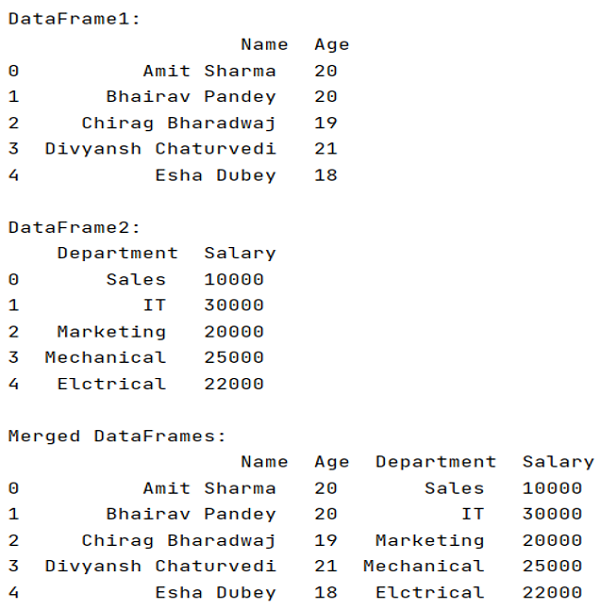# How to merge two DataFrames by index?

Given two DataFrames, we have to merge them by index. By Pranit Sharma Last updated : September 20, 2023

DataFrames are 2-dimensional data structures in pandas. DataFrames consist of rows, columns, and the data. DataFrame can be created with the help of python dictionaries or lists but in the real world, CSV files are imported and then converted into DataFrames. Sometimes, DataFrames are first written into CSV files. Here, we are going to merge two DataFrames by index.

## Problem statement

Given two DataFrames, we have to merge them by index.

## Merging two DataFrames by index

For this purpose, we will use pandas.DataFrame.merge() method. When we want to update a DataFrame with the help of another DataFrame, we use df.merge() method. This method is used to merge two DataFrames based on an index.

Syntax:

```DataFrame.merge(
right,
how='inner',
on=None,
left_on=None,
right_on=None,
left_index=False,
right_index=False,
sort=False,
suffixes=('_x', '_y'),
copy=True,
indicator=False,
validate=None
)
```

Let us understand with the help of an example.

## Python program to merge two DataFrames by index

```# Importing pandas package
import pandas as pd

# Creating a Dictionary
dict1 = {
'Name':['Amit Sharma','Bhairav Pandey','Chirag Bharadwaj','Divyansh Chaturvedi','Esha Dubey'],
'Age':[20,20,19,21,18]
}

dict2 = {
'Department':['Sales','IT','Marketing','Mechanical','Elctrical'],
'Salary':[10000,30000,20000,25000,22000]
}

# Creating a DataFrame
df1 = pd.DataFrame(dict1)
df2 = pd.DataFrame(dict2)

# Display DataFrame
print("DataFrame1:\n",df1,"\n")
print("DataFrame2:\n",df2,"\n")

# Merging two DataFrames
result = pd.merge(df1,df2,left_index=True, right_index=True)

# Display Result
print("Merged DataFrames:\n",result)
```

Here, we have passed two parameters, left_index and right_index which generally means whether to use the index from left and right DataFrame or not, we want the DataFrames to be merged on the basis of index, hence we will set left and right index as True.

### Output

The output of the above program is: